# Role of Operator Precedence Parser

In this, we will cover the overview of Operator Precedence Parser and mainly focus on the role of Operator Precedence Parser. And will also cover the algorithm for the construction of the Precedence function and finally will discuss error recovery in operator precedence parsing. Let’s discuss it one by one.

Introduction :
Operator Precedence Parser constructed for operator precedence grammar.  Operator precedence grammar is a grammar that doesn’t contain epsilon productions and does not contain two adjacent non-terminals on R.H.S. of any production. Operator precedence grammar is provided with precedence rules. Operator Precedence grammar could be either ambiguous or unambiguous.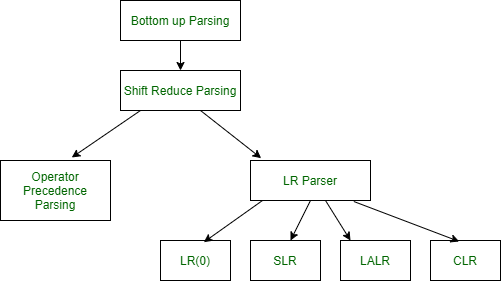Operator Precedence Parser Algorithm :

```1. If the front of input \$ and top of stack both have \$, it's done
else
2. compare front of input b with ⋗
if b! = '⋗'
then push b
scan the next input symbol
3. if b == '⋗'
then pop till ⋖ and store it in a string S
pop ⋖ also
reduce the popped string
if (top of stack) ⋖ (front of input)
then push ⋖ S
if (top of stack) ⋗ (front of input)
then push S and goto 3 ```

Components Operator Diagram :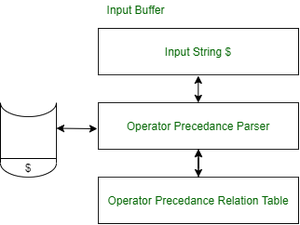Components Operator

Example –
Let’s take an example to understand the role of operator precedence as follows.

```E-> E+T/T
T-> T*V/V
V->a/b/c/d
string= "a+b*c*d"```

Implementation of the above algorithm for the string “a+b*c*d” as follows.

Algorithm for construction of Precedence function :

1. Generate a function Xa for each grammar terminal a and for the end of the string symbol.
2. Partition the symbol in groups so that Xa and Yb are the same groups if a ≐ b.
3. Generate a directed graph whose nodes are in the groups, for each symbol a and b, do place an edge from the group of Yb to the group of Xa if a ⋖ b, otherwise if a ⋗ b place an edge from the group of Xa to that of Yb.
4. If the constructed graph has a cycle then no procedure functions exist. When there are no cycles collect the length of the longest paths from the groups of Xa and yb respectively.

Example –
Let’s take an example to understand the construction of precedence function as follows.

`E -> E + E/E * E/( E )/id`

Here, you will see the Operator precedence relation table and Precedence Relations Graph diagram. Let’s have a look.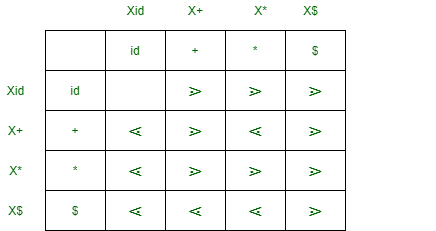Operator precedence relation table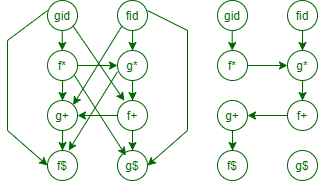Precedence Relations Graph

As we can see no cycle there is no cycle in the graph, we can make this function table as follows.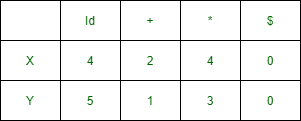Function table

Columns Representation function :

Columns represent function Ya and Rows represent function Xa –
It is calculated by taking the longest path from Xid to X\$ and Yid to Y\$.

```fid -> g* -> f+ ->g+ -> f\$
gid -> f* -> g* ->f+ -> g+ ->f\$ ```
• The disadvantage of the operator precedence relation table is that if there are ‘n’ numbers of symbols then we require a table of n*n to store them. On other hand, by using the operator function table, to accommodate n number of symbols we require a table of 2*n. Operator Precedence Grammar cannot decide the unary minus(lexical analyzer should handle the unary minus).
• The advantage of using Operator Precedence Grammar is simple and enough powerful for expression in programming languages.

Error Recovery in Operator-Precedence Parsing :

• Error Cases –
1. No relation holds between the terminal on the top of the stack and the next input symbol.
2. A handle is found (reduction step), but there is no production with this handle as a right side.

• Error recovery –
1. Each empty entry is filled with a pointer to an error routine.
2. Decides the popped handle “looks like” which right-hand side. And tries to recover from that situation.

• Handling shift/ reduce errors –
To take care of such types of errors we must modify the following.
1. Stack
2. Input
3. or Both